Computational & Technology Resources an online resource for computational,engineering & technology publications not logged in - login Civil-Comp ProceedingsISSN 1759-3433 CCP: 83PROCEEDINGS OF THE EIGHTH INTERNATIONAL CONFERENCE ON COMPUTATIONAL STRUCTURES TECHNOLOGY Edited by: B.H.V. Topping, G. Montero and R. Montenegro Paper 106A Mixed Finite Element Model based on Least-Squares Formulation for the Static Analysis of Laminated Composite Plates F. Moleiro1, C.M. Mota Soares1, C.A. Mota Soares1 and J.N. Reddy21IDMEC/IST, Department of Mechanical Engineering, Instituto Superior Técnico, Technical University of Lisbon, Portugal 2Department of Mechanical Engineering, Texas A&M University, College Station, Texas, United States of America doi:10.4203/ccp.83.106 Full Bibliographic Reference for this paper F. Moleiro, C.M. Mota Soares, C.A. Mota Soares, J.N. Reddy, "A Mixed Finite Element Model based on Least-Squares Formulation for the Static Analysis of Laminated Composite Plates", in B.H.V. Topping, G. Montero, R. Montenegro, (Editors), "Proceedings of the Eighth International Conference on Computational Structures Technology", Civil-Comp Press, Stirlingshire, UK, Paper 106, 2006. doi:10.4203/ccp.83.106 Keywords: mixed finite elements, least-squares, laminated composite plates. Summary This paper presents a mixed finite element model based on least-squares variational principles, as an alternative approach to the mixed weak form finite element models. The mixed least-squares model considers the static analysis of laminated composite plates using the first-order shear deformation theory, with generalized displacements and stress resultants as independent variables. In fact, the mixed model is developed using equal-order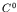Lagrange interpolation functions with high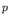-levels, along with full integration. The motivation for this finite element model comes from the previous works of Pontaza and Reddy , Pontaza  and also, Duan and Lin . Their recent mixed finite element formulations based on least-squares variational principles have shown promising theoretical and computational advantages, altogether in solid and in fluid mechanics. Actually, Pontaza and Reddy  developed a mixed element based on least-squares formulation for the bending of single-layered isotropic plates, using the classical plate theory (CLT) and first-order shear deformation theory (FSDT). As an extension of that work to the static analysis of laminated composite plates, a mixed least-squares FSDT finite element model is here introduced. The real benefit of least-squares variational principles combined with mixed formulations is that it leads to a variational unconstrained minimization problem, where the finite element approximation spaces can be chosen independently. Therefore, no restrictive compatibility conditions on the discrete spaces arises, contrary to the stability requirements intrinsic to mixed weak form finite element models. In addition, the mixed least-squares-based discrete model, once the boundary conditions are duly imposed, yields a symmetric positive-definite system of algebraic equations, instead of the indefinite system in mixed weak form models. In overview, this paper addresses first the governing equations consistent with the mixed formulation of the proposed least-squares finite element model. Basically, the governing equations consist of the plate equilibrium equations along with the laminate constitutive equations, as detailed in Reddy . Secondly, the subsequent least-squares formulation is presented and the finite element model derived. In essence, the least-squares functional is defined by measuring the residuals of the governing equations in terms of suitable norms. Then, the finite element model is developed by minimizing the least-squares functional with respect to the chosen approximation spaces. In fact, it should be noted that the use of high-order interpolation functions and full integration are the appropriate way to truly minimize the least-squares functional. Pontaza and Reddy  and later Pontaza  demonstrated the exponential fast decay of the least-squares functional with increasing order of the element. Lastly, numerical examples to assess the predictive capabilities of the proposed mixed least-squares model are considered. Static analysis results for four laminated composite plates with different boundary conditions and a series of side-to-thickness ratios, ranging from thick to thin laminates, are thoroughly examined. For comparison, analytical solutions using FSTD by the well-known Navier and Lévy methods described in Reddy  are presented alongside the numerical results. Each laminate considered, is systematically modelled using either 4th, 6th or 8th-order elements in uniform meshes of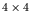,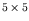or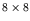elements. Overall, the numerical results show excellent agreement with the analytical solutions, for the entire side-to-thickness ratios investigated. In fact, the proposed mixed least-squares model with high-order interpolation functions is shown to be insensitive to shear-locking. Most especially, convergence of the computed results towards the analytical solutions is verified for both- and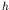-refinements. References 1 J.P. Pontaza, J.N. Reddy, "Mixed Plate Bending Elements based on Least-Squares Formulation", International Journal for Numerical Methods in Engineering, 60, 891-922, 2004. doi:10.1002/nme.991 2 J.P. Pontaza, "Least-Squares Variational Principles and the Finite Element Method: Theory, Formulations, and Models for Solid and Fluid Mechanics", Finite Elements in Analysis and Design, 41, 703-728, 2005. doi:10.1016/j.finel.2004.09.002 3 H.-Y. Duan, Q. Lin, "Mixed Finite Elements of Least-Squares Type for Elasticity", Computer Methods in Applied Mechanics and Engineering, 194, 1093-1112, 2005. doi:10.1016/j.cma.2004.06.033 4 J.N. Reddy, "Mechanics of Laminated Composite Plates and Shells - Theory and Analysis", CRC Press, Boca Raton, 2004. purchase the full-text of this paper (price £20) Back to top ©Civil-Comp Limited 2022 - terms & conditions